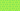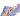PricingResources

# What Are Regression Statistics?

Written by

The analysis of regression statistics is a process undertaken in financial modeling to identify trends in data and measure the relationship between two separate variables. In finance, regression analysis typically involves analyzing and quantifying the impact of an independent variable on a dependent variable.

By knowing how different independent variables affect dependent variables, you’re able to better plan financially for the future.

What are dependent and independent variables in regression statistics?

In finance, dependent variables are attributes that change when influenced by independent variables, like revenue, for example. The dependent variable is whatever you’re trying to measure; it doesn’t have to be related to sales or earnings, it could even be something like customer satisfaction.

On the other hand, an independent variable is any attribute that, when changed, will impact a dependent variable. For example, number of staff is an independent variable, as increasing or decreasing the number of staff you’re paying will directly impact your revenue – a dependent variable.

An independent variable can be anything that would alter a dependent variable, like weather affecting how much you make from in-store sales, customer demographics affecting how many new customers you’re bringing in, a recession impacting how many returning customers you have, and so on.

To put it in layman’s terms, imagine you’re reviewing a movie. Your overall rating of the movie is the dependent variable, while anything from casting to writing to direction to the length of the movie itself can be independent variables, as any of these factors can impact how you rate it.

Regression analysis is the process of measuring the relationship between independent and dependent variables. There are a few types of regression analysis, with the most common being linear regression and multiple linear regression. The process typically involves using data to form a regression model graph.

Regression analysis is similar in objectives to sensitivity analysis. Analysis of variance (ANOVA) is another process used to compare variables and their relationship.

## How to do regression analysis

You’ll first define a single dependent variable you’re looking to measure and then hypothesize which independent variable or variables you feel may influence it.

You’ll then need to gather the data. Depending on what factors you’re assessing, this could mean conducting research with customer surveys, or it could be looking at your own existing data if you wanted to, for example, see how changing the price of a product impacts the number of sales you make.

Then, you’ll plot the data on a scatter graph. With our pricing example, the number of sales (the dependent variable) would form the y-axis while the price of the product will form the x-axis. You’ll then be able to start identifying trends and correlations.

In order to quantifiably grade the relationship between the two, you’ll want to next draw a line through the middle of the data points – this is called the regression line. It helps to use a regression analysis formula on a program like Excel to make sure the regression line is accurate. If your data points don’t appear to fit any clear line, this might mean there’s not a whole lot of correlation between your dependent and independent variables.

The simple equation is:

Y represents the dependent variable, X is the independent variable, b is the slope of the regression line, a is the y-intercept (the point where the graph crosses the y-axis), and ϵ represents residual (error).

While the above applies to simple linear regression, if you wanted to see the impact of several independent variables at once, you’d need to use multiple linear regression.

The equation for multiple linear regression is:

Values b, c, and d represent slopes, while the remaining values are the same as in simple linear regression. Multiple linear regression helps you identify which independent variables have the strongest impact on the dependent variable.

## When to use regression analysis

Regression analysis can be used to forecast revenues and expenses based on various factors. For example, you can use regression analysis to predict your revenue in five years if you were to increase your prices by the same amount each year. After performing a regression analysis, you’ll have a more accurate, data-driven idea of which factors are most important, which areas need improvement, and which areas are doing you good.

#### We can help

GoCardless helps you automate payment collection, cutting down on the amount of admin your team needs to deal with when chasing invoices. Find out how GoCardless can help you with ad hoc payments or recurring payments.## Interested in automating the way you get paid? GoCardless can help

Contact sales

Sales

Contact Sales

+1(415) 523-2279

Support

help@gocardless.com

+1 (628) 241-0044